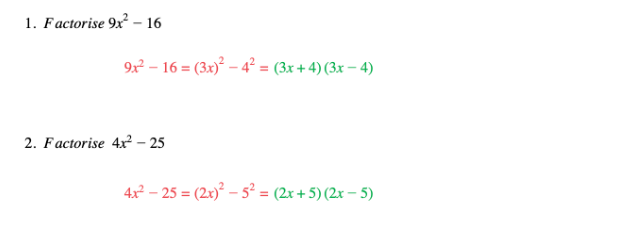# Edexcel IGCSE Maths 复习笔记 2.4.3 Difference of Two Squares

Edexcel IGCSE Maths 复习笔记 2.4.3 Difference of Two Squares

#### What is the difference of two squares?

• A “Difference Of Two Squares” is any expression in the form p2 – q2

#### Why is it so important?

• It comes up a lot – recognising it can save a lot of time

#### How does it factorise?

• p2 – q2 can always be factorised in the same way:p2 – q2 = (p + q)(p – q)

(Try multiplying it out to see why…)

#### Worked Example